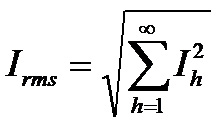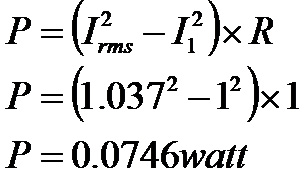As a continuation of my article on effects of harmonics on electrical equipment, let us provide an example of the effects of harmonic currents on 1-ohm resistive load drawing 1 ampere fundamental current containing 5th, 7th, 11th & 13th harmonic currents. Ideally, the amplitudes of harmonic currents are inversely proportional to their harmonic orders.

The rms current can be calculated as followsFrom effects of harmonics on electrical equipment the magnitude of harmonics currents are:

5th Harmonic (250 Hz): 20.0% x I1
7th Harmonic (350 Hz): 14.3% x I1
11th Harmonic (550 Hz): 9.1% x I1
13th Harmonic (650 Hz): 7.7% x I1

where: I1 is the fundamental current which is 1 A as per our example.It is very clear that the current increased by 3.7% compared to a pure sinusoidal current.

The increase in power due to harmonics will beThis is a 7.46% increase in power consumption.

Assuming that you are using an 2000W electric heater in this system, your additional power consumption will be 2000 x 0.0746 = 149.2 watts. If you are using this for about 3 hours a day during the winter months, that will be
= 149.2 watts x 3 hrs x 30 days x 3 months
= 40,284 watt-hours or 40.28 kwh.

Categories:

Tags: